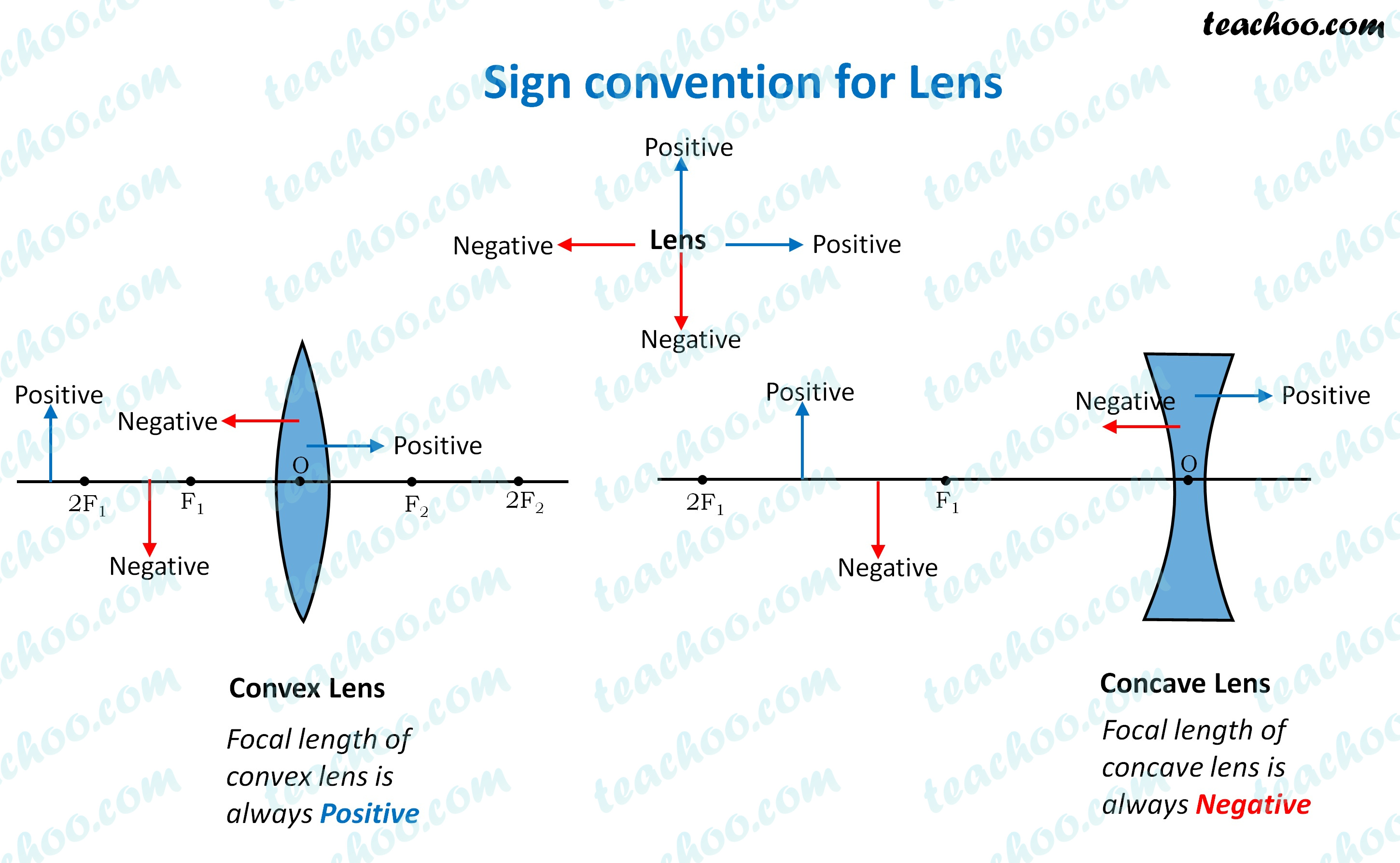Concepts

Class 10
Chapter 10 Class 10 - Light - Reflection and Refraction

In numericals, we need to calculate some things like

• Object distance
• Image distance
• Object height
• Image height
• Focal length

To calculate them correctly, we need to find whether it is positive or negative

The sign convention mentioned below helps us in itWe can see the sign convention in both Lens

Some points to note

• Focal length of Convex Lens is positive
• Focal length of Concave Lens is negative
• Since object is always in front of the lens, object distance is always negative
• If a real image is formed, image is formed on right side, so image distance is positive
• If a virtual image is formed, image is formed on left side, so image distance is negative
• Since object is always above the principal axis, object height will be positive
• If image is above the principal axis, image height will be positive. It means that image is erect
• If image is below the principal axis, image height will be negative. It means that image is inverted

Learn in your speed, with individual attention - Teachoo Maths 1-on-1 Class